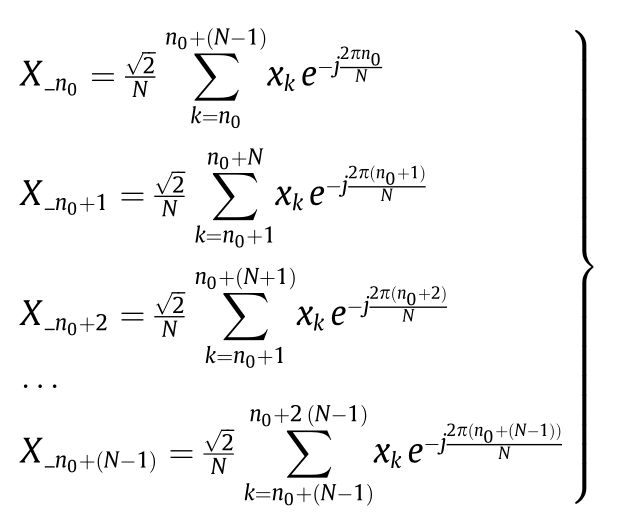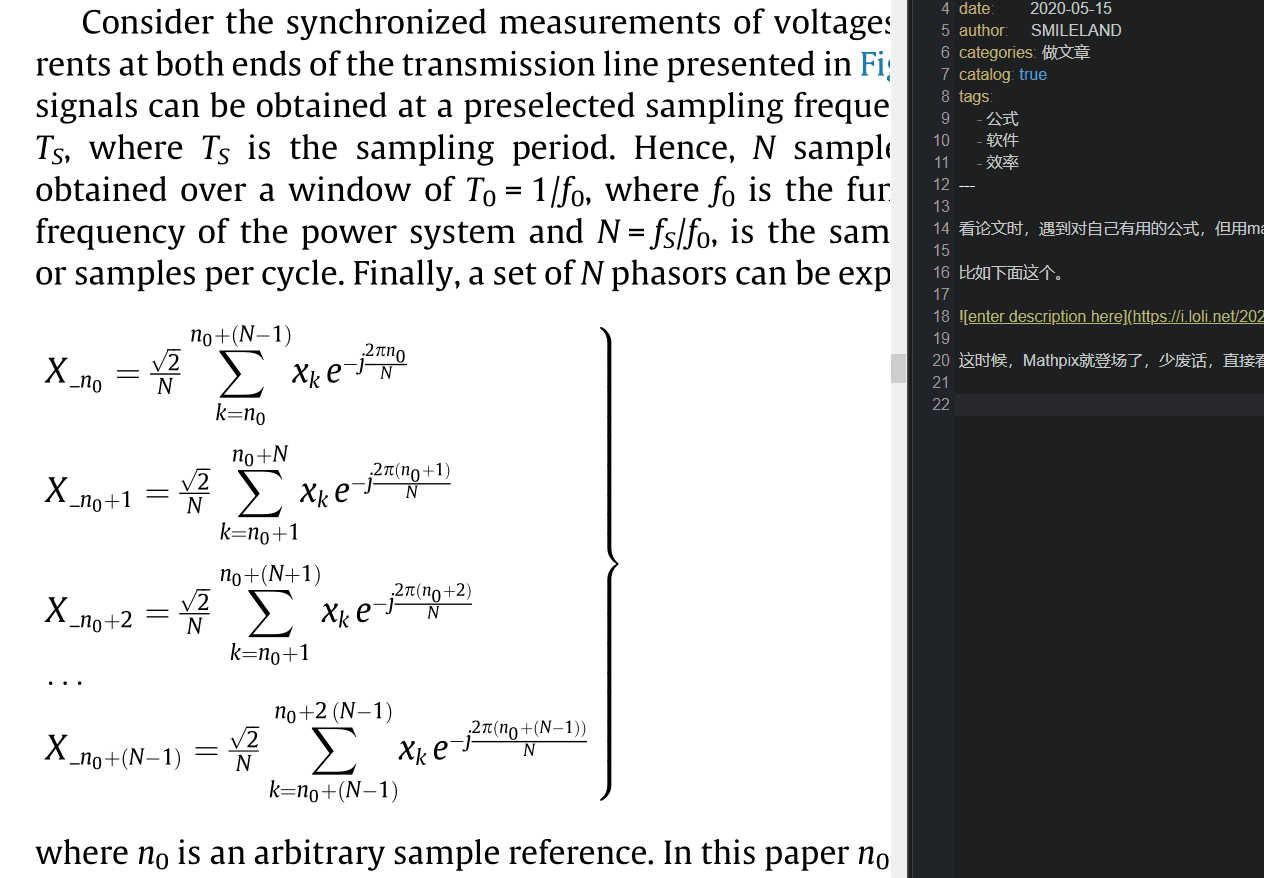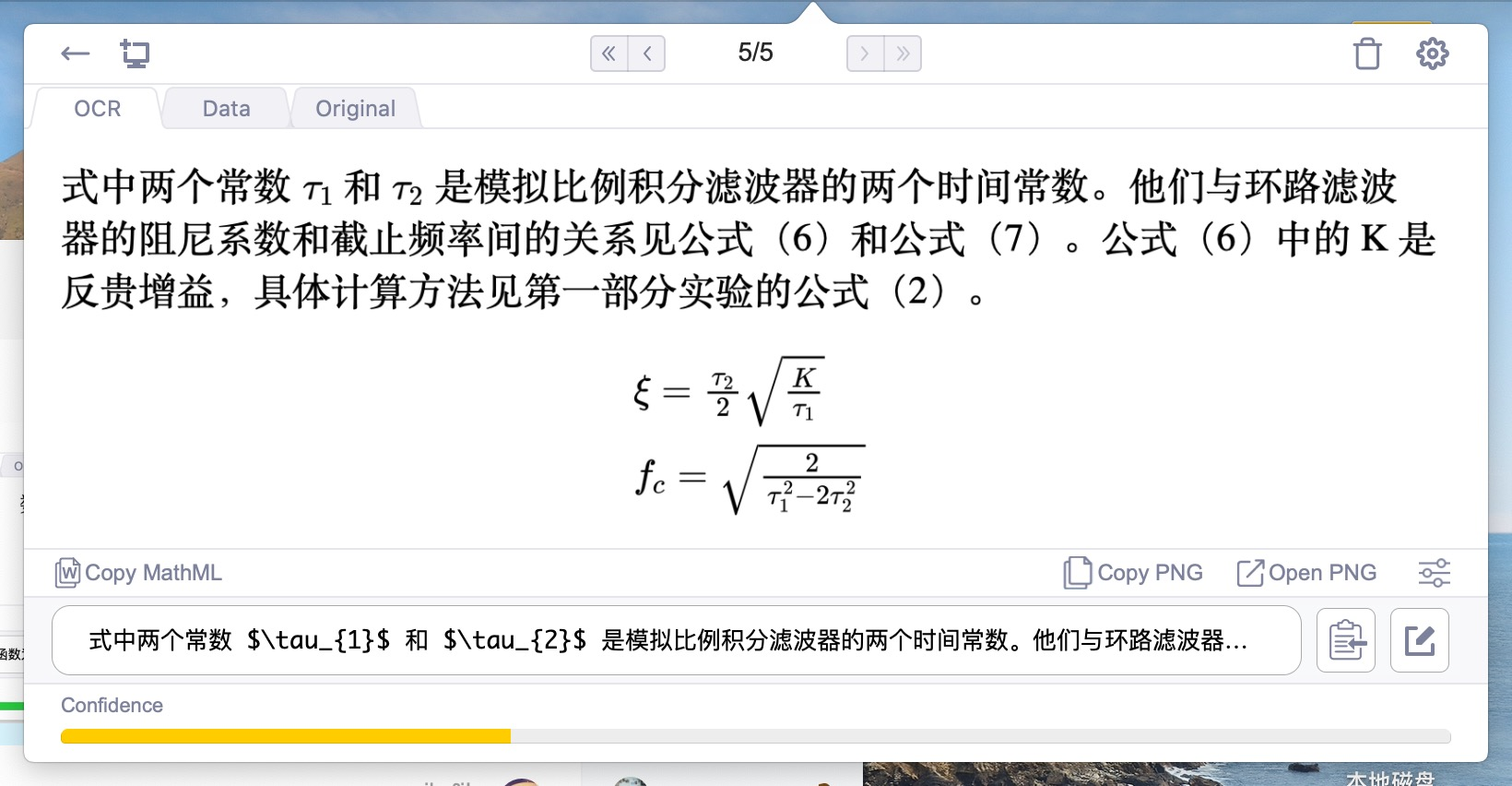LaTeX是一个排版系统，是一个只用代码进行排版的系统，但这里只是借用了LaTeX中对公式的编码部分，举个例子。

$U_a$


$U_a$

$U=I^2*R$


$U=I^2*R$

Mathpix就是一款首先将需要识别的公式截图，然后对其进行OCR识别，自动转换成为LaTeX代码的软件，由于LaTeX代码是一种统一编码规则，Mathtype也是支持的，直接将LaTeX代码复制进Mathtype输入框会自动还原成直观显示方式，并且可以进行修改。式中两个常数 $\tau_{1}$ 和 $\tau_{2}$ 是模拟比例积分滤波器的两个时间常数。他们与环路滤波

$\begin{array}{l} \xi=\frac{\tau_{2}}{2} \sqrt{\frac{K}{\tau_{1}}} \\ f_{c}=\sqrt{\frac{2}{\tau_{1}^{2}-2 \tau_{2}^{2}}} \end{array}$



https://mathpix.com/